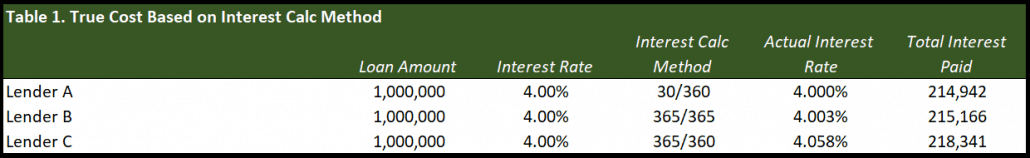# 30/360, Actual/365, and Actual/360 – How Lenders Calculate Interest on CRE Loans – Some Important Insights(Updated August 7, 2019 to include a Watch Me Build video and Downloadable file)

Commercial real estate lenders commonly calculate loans in three ways: 30/360, Actual/365 (aka 365/365), and Actual/360 (aka 365/360). Real estate professionals should be aware of these methods if they want to understand the real interest rate as well as the total amount of interest being paid over the term of a loan.

This post will go over how these calculations are commonly done.

## Overview of Lender Interest Calculation

If three different lenders are offering identical loans with identical loan amounts and interest rates, but each lender is using one of the three interest calculations, a borrower will pay the least interest with 30/360 and the most with Actual/360.

### An example

To give an example, let say the three lenders are each offering a \$1,000,000 loan at 4% for 10 years with no interest only period. Lender A is using 30/360, lender B is using Actual/365, and lender C is using Actual/360. The actual interest rate and total payments are shown for each lender below:As the table shows, Lender A has the most favorable terms for a borrower and Lender C has the least favorable. The next section will explain these calculations using our example above.

Down at the bottom of this post is a Watch Me Build video and downloadable file where you can follow along and build the amortization tables in these examples with me.

#### 30/360

30/360 is calculated by taking the annual interest rate proposed in the loan (4%) and dividing it by 360 to get the daily interest rate (4%/360 = 0.0111%). Then, take the daily interest rate and multiply it by 30 to get the monthly interest rate (0.333%). This loan calculation assumes that there are 360 days a year and 30 days in each month. This interest calculation method returns a true 4% interest rate.

Another way to look at this is by simply taking the 4% interest rate and multiplying it by (30/360). 30/360 reduces to 1/12, so we can really just divide 4% by 12.

#### Actual/365 (aka 365/365)

Actual/365 is calculated by taking the annual interest rate and dividing it by 365 and then multiplying that number by the amount of days in the current month. For example, if we are in the month of February, we would take the 4% interest rate and divide it by 365 to get 0.0110%, then multiply it by 28 (29 on a leap year, hence why the word actual and the number 365 are interchangeable in the name) to get 0.307% (0.318% on a leap year).

Take note that although dividing the annual interest rate by 365 results in a smaller daily interest rate, the fact that we multiply by the actual days in each month as opposed to 30, ultimately results in an overall slightly larger amount of interest paid when compared to the 30/360 method due to the extra day in a leap year.

#### Actual/360 (aka 365/360)

This method has seen its day in court because borrowers challenged that this method is deceptive and hides from borrowers the true cost of borrowing. However, the lenders prevailed due to the fact that it was fully disclosed how they are calculating interest. Thus, Actual/360 is an interest calculating method that is here to stay.

When using the Actual/360 method, the annual interest rate is divided by 360 to get the daily interest rate and then multiplied by the days in the month. This creates a larger dollar amount in interest payments because dividing the annual rate by 360 creates a larger daily rate then dividing it by 365.

So, essentially the annual interest rate is divided by 360 (larger than dividing by 365) then multiplied by 365 or 366 in a leap year. Both 365/360 and 365/365 interest calculation methods are shown side by side in the table below:In Table 1, the stated interest rates for 365/365 and 365/360 is 4.003% and 4.058%, respectively. This is because in Table 1, 3 years of interest payments are calculated by multiplying the daily rate by 365 and 1 year is calculated by multiplying the daily rate by 366. These four calculations are then divided by 4.

Note: Always defer to the loan documentation and review the explicit methodology for how interest will be calculated. The terminologies used may mean slightly different things to different people, but the documentation will always be explicit in how the interest will be calculated.